## Dynamic Discrete Equivalent Model of Photovoltaic Power Generation System

LIU Kezhen, CHEN Xueou, CHEN Leidan, LIN Zheng, SHEN Fu,

School of Electric Power Engineering, Kunming University of Science and Technology, Kunming 650500, China

 基金资助: 国家自然科学基金(52107097)云南省应用基础研究计划(202101BE070001-061)云南省应用基础研究计划(202201AU070111)昆明理工大学高层次人才平台建设(KKZ7202004004)

Received: 2021-12-10   Revised: 2022-03-6   Accepted: 2022-05-5Abstract

The proportion of renewable energy in the new power system is further increased, and the grid connected capacity of photovoltaic units has a trend of obvious improvement. The dynamic behavior of the photovoltaic (PV) power generation system at different permeabilities has a significant impact on the load characteristics of the power grid. However, the complex dynamic model of photovoltaic power generation grid connection and the large number of parameters to be identified increase the difficulty of practical application of the model. Therefore, a dynamic discrete equivalent model of the PV power generation model based on the physical model of the PV power generation model is established, and the parameters of the dynamic discrete equivalent model for the PV power generation model are obtained. The IEEE 14-bus system, which is subject to various PV permeabilities, is adopted to verify the superb dynamic characteristics of the proposed discrete equivalent model for the PV power generation in power system simulations. The pertinent simulation results show that the dynamic discrete equivalent model of the PV power generation system can accurately describe the dynamic characteristics of the PV power generation system with a high accuracy and an easy identification performance.

Keywords： photovoltaic (PV); dynamic equivalence; various permeabilities; parameter identification

LIU Kezhen, CHEN Xueou, CHEN Leidan, LIN Zheng, SHEN Fu. Dynamic Discrete Equivalent Model of Photovoltaic Power Generation System[J]. Journal of Shanghai Jiaotong University, 2023, 57(4): 412-421 doi:10.16183/j.cnki.jsjtu.2021.505

## 1 光伏发电系统并网动态模型

### 图1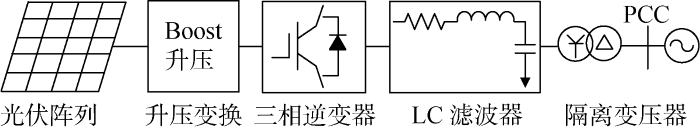Fig.1   Grid connected model of photovoltaic power generation system

$P=3(ugdId+ugqIq)/2Q=-3(ugqId-ugdIq)/2$

$ugq$为0,则:

$P=3ugdId/2Q=3ugdIq/2$

### 图2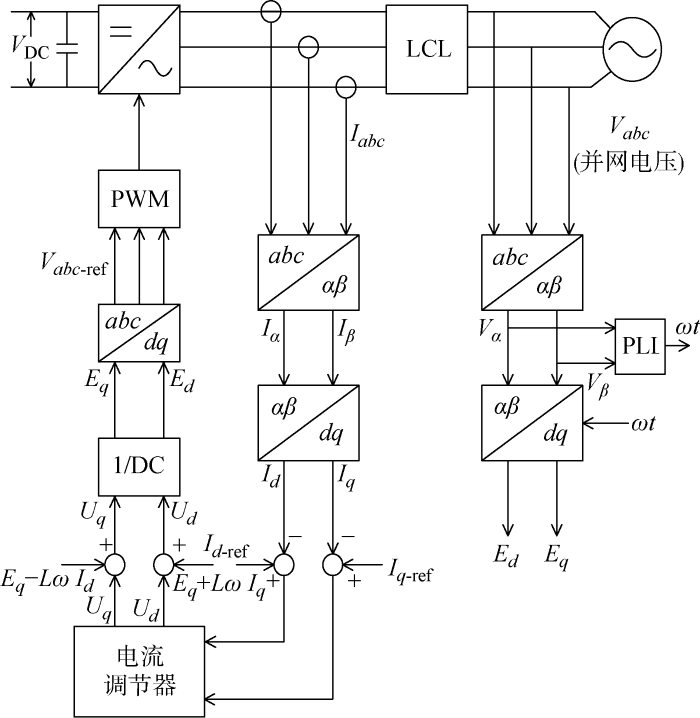Fig.2   Grid connected control topology of photovoltaic power generation system

## 2 光伏发电系统动态离散等值模型

### 图3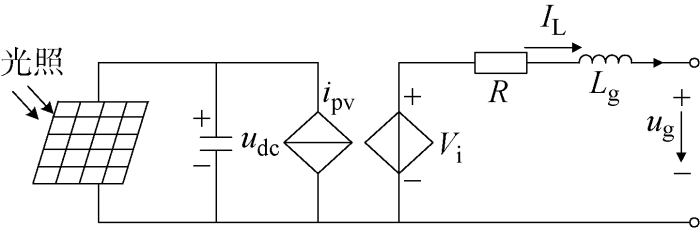Fig.3   Single phase dynamic equivalent model of photovoltaic power generation system

$dIddt=1L(uid-ugd-IdR)-ωIqdIqdt=1L(uiq-ugq-IdR)+ωIddudcdt=1C(ipv-1.5SdId-1.5SqIq)$

$ΔId(s)ΔIq(s)=f1(s)f2(s)f3(s)f4(s)ΔUgd(s)ΔUgq(s)$

$f1(s)=B11s3+B12s2+B14sA11s4+A12s3+A13s2+A14s$
$f2(s)=B13s2+B15sA11s4+A12s3+A13s2+A14s$
$f3(s)=B22s2+B25sA11s4+A12s3+A13s2+A14s$
$f4s=B21s3+B23s2+B24sA11s4+A12s3+A13s2+A14s$

,,,,,,,

$ΔIr(s)ΔUg(s)=Ars3+Crs2+BrsA11s4+A12s3+A13s2+A14sΔIj(s)ΔUg(s)=Ajs3+Cjs2+BjsA11s4+A12s3+A13s2+A14s$

$Ar=sin2θ02(B21-B11)+sin2θ0B11+cos2θ0B21$
$Aj=sin2θ02(B21-B11)+sin2θ0B21+cos2θ0B11$

θ0为并网功率因数角.

$ΔIr(k+4)=θα1ΔIr(k+3)+ θα2ΔIr(k+2)+θα3ΔIr(k+1)+ θα4ΔIr(k)+θα5ΔU(k+4)+ θα6ΔU(k+3)+θα7ΔU(k+2)+ θα8ΔU(k+1)+θα9ΔU(k)ΔIj(k+4)=θβ1ΔIj(k+3)+ θβ2ΔIj(k+2)+θβ3ΔIj(k+1)+ θβ4ΔIj(k)+θβ5ΔU(k+4)+ θβ6ΔU(k+3)+θβ7ΔU(k+2)+ θβ8ΔU(k+1)+θβ9ΔU(k)$

## 3 光伏发电系统动态离散等值模型参数辨识方法

$yi=f(xi,θ), i=1,2,…,m$

$x1=ΔIr(k+3)$,,,,,,

,,,,,,

,,,,,,

,

$J=∑i=1mεi2=εTε=[yi-f(xi,θ)]T[yi-f(xi,θ)]$

$θ^=(xTixi)-1xTiy'i$

$E=∑k=1N(Irk-I^rk)2N$
$E^=∑k=1N(Ijk-I^jk)2N$

## 4 仿真验算及分析

### 图4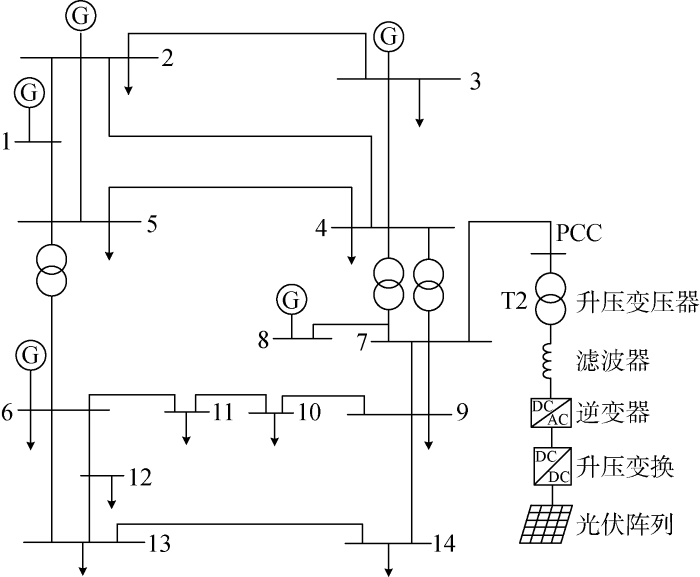Fig.4   Photovoltaic power generation system integrated into IEEE 14-bus topology

(1) 输电系统参数.

IEEE 14节点中基准电压为23 kV,系统频率为50 Hz,系统阻抗采用串联型RL,参数分别为电感0.618 H、串联电阻0.4 Ω.

(2) 配电系统参数.

Tab.1  Parameters of photovoltaic cell

(3) 算例内容.

(1) 模型普适性验证.

### 图5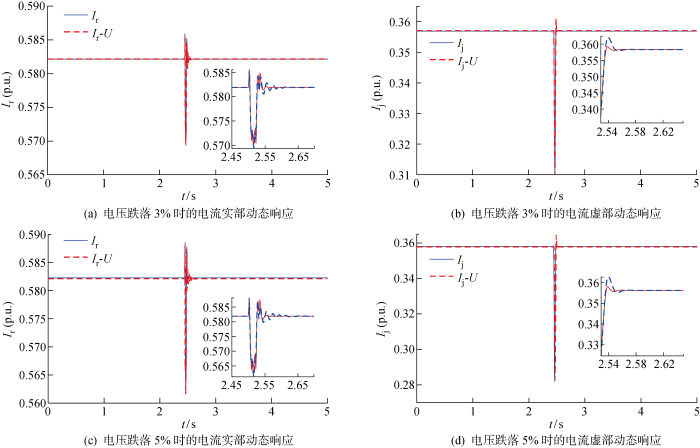Fig.5   Dynamic response description of three-phase fault with a permeability of 30%

### 图6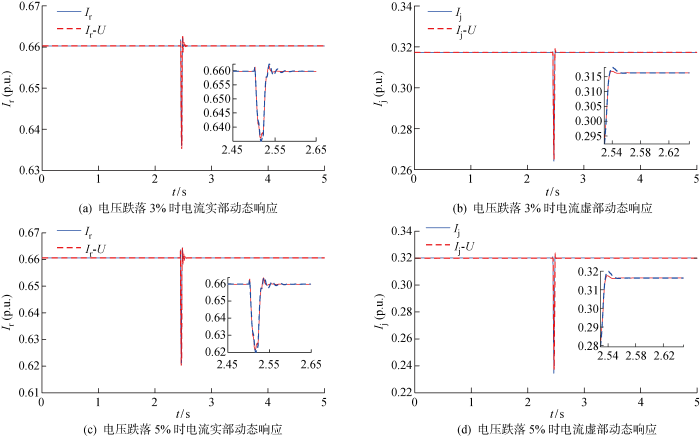Fig.6   Dynamic response description of three-phase fault with a permeability of 60%

### 图7Fig.7   Dynamic response description of single-phase fault with a permeability of 20%

### 图8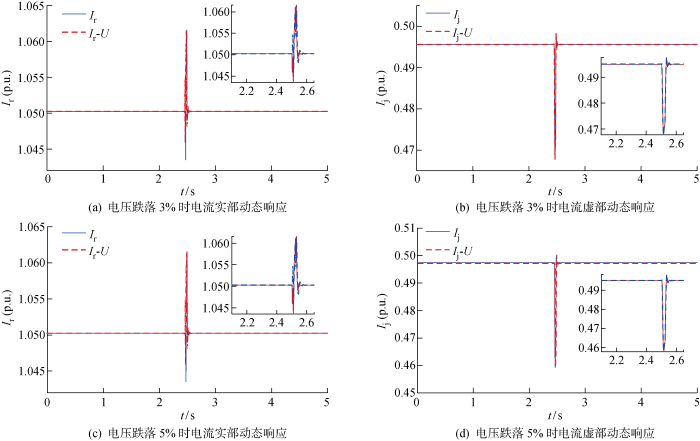Fig.8   Dynamic response description of single-phase fault with a permeability of 40%

(2) 步长影响性能分析.

Tab.2  Fitting results of current parameters in three-phase short-circuit grounding fault at different permeabilities

0.0013031.099×10-41.502×10-4
0.0013052.044×10-42.647×10-4
0.0016031.056×10-41.003×10-4
0.0016052.089×10-42.389×10-4
0.0023031.517×10-49.681×10-5
0.0023052.292×10-41.926×10-4
0.0026038.541×10-58.792×10-5
0.0026051.535×10-42.154×10-4

(3) 模型适用范围研究.

### 图9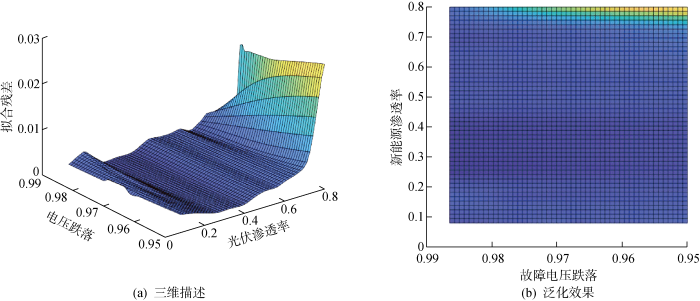Fig.9   Generalization ability of the model with a voltage drop depth less than 5% at different permeabilities

### 图10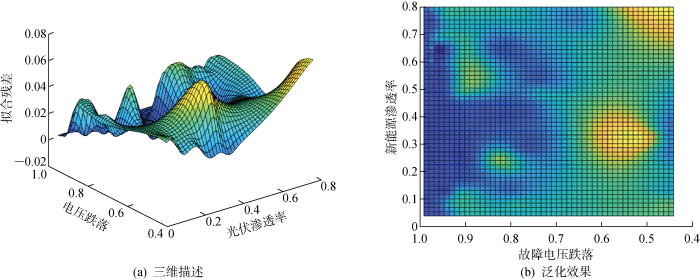Fig.10   Generalization ability of the model with a voltage drop depth less than 40% at different permeabilities

## 5 结论

(1) 基于光伏电站机理模型,建立了光伏并网发电系统的动态4阶离散等值模型,获取动态离散等值模型的模型参数,并采用最小二乘法辨识模型参数,研究发现所提模型精度高、易于辨识.

(2) 从模型的普适性、步长对模型仿真的性能影响、模型的适用范围验证了所提模型的准确性.同时,经过辨识发现模型参数间存在一定规律,可将其作为参数是否辨识正确的依据.

(3) 相较于渗透率,电压跌落的深度对模型泛化影响更加显著.

## 附录A

$θα1=16A13h2+16A12h+64A112A14h3+4A13h2+8A12h+16A11$
$θα2=24A13h2+96A112A14h3+4A13h2+8A12h+16A11$
$θα3=-16A13h2+16A12h-A112A14h3+4A13h2+8A12h+16A11$
$θα4=-2A14h3-4A13h2+8A12h-16A112A14h3+4A13h2+8A12h+16A11$
$θα5=2Brh3+4C4h2+8Arh2A14h3+4A13h2+8A12h+16A11$
$θα6=-16Arh2A14h3+4A13h2+8A12h+16A11$
$θα7=-12Crh22A14h3+4A13h2+8A12h+16A11$
$θα8=-4Brh3+24Arh2A14h3+4A13h2+8A12h+16A11$
$θα9=2Brh3-4Crh2-8Arh2A14h3+4A13h2+8A12h+16A11$
$θβ1=16A13h2+16A12h+64A112A14h3+4A13h2+8A12h+16A11$
$θβ2=24A13h2+96A112A14h3+4A13h2+8A12h+16A11$
$θβ3=-16A13h2+16A12h-A112A14h3+4A13h2+8A12h+16A11$
$θβ4=-2A14h3-4A13h2+8A12h-16A112A14h3+4A13h2+8A12h+16A11$
$θβ5=2Bjh3+4Cjh2+8Ajh2A14h3+4A13h2+8A12h+16A11$
$θβ6=-16Ajh2A14h3+4A13h2+8A12h+16A11$
$θβ7=-12Cjh22A14h3+4A13h2+8A12h+16A11$
$θβ8=-4Bjh3+24Ajh2A14h3+4A13h2+8A12h+16A11$
$θβ9=2Bjh3-4Cjh2-8Ajh2A14h3+4A13h2+8A12h+16A11$

Tab.A1  Identification results of current real part parameters in three-phase fault

/%

θα1θα2θα3θα4θα5$∑i=15$θαiθα6θα7θα8θα9$∑i=69$θαi
032.1553-2.14871.4263-0.5645-0.26920.60010.6795-0.63890.4571-0.18500.3127
052.2664-2.34751.5473-0.5854-0.28960.59120.7701-0.77500.5368-0.20600.3259
6031.8282-1.40170.8280-0.3939-0.17820.68240.5087-0.36090.1878-0.05190.2837
6051.8644-1.46690.8745-0.4106-0.19440.66700.5544-0.42320.2256-0.06390.2929

Tab.A2  Identification results of virtual part of current in three-phase fault

/%

θβ1θβ2θβ3θβ4θβ5$∑i=15$θβiθβ6θβ7θβ8θβ9$∑i=69$θβi
3031.2533-0.57390.6167-0.41720.12681.0057-0.05330.06180.0724-0.00860.0723
3051.3851-0.86030.8250-0.47910.14791.0186-0.16310.2012-0.02810.03540.0454
6031.2803-0.91831.0121-0.51620.052370.910270.04690.3631-0.1369-0.07320.1999
6051.3367-0.96570.9922-0.50250.04960.9103-0.00090.4329-0.2098-0.04190.1803

## 参考文献 原文顺序 文献年度倒序 文中引用次数倒序 被引期刊影响因子

[J]. 上海交通大学学报, 2022, 56(5): 554-563.

LI Linyan, HAN Shuang, QIAO Yanhui, et al.

A wind-solar-electric vehicles coordination scheduling method for high proportion new energy grid-connected scenarios

[J]. Journal of Shanghai Jiao Tong University, 2022, 56(5): 554-563.

[J]. 电力自动化设备, 2020, 40(5): 1-9.

WU Feng, DAI Xiaomei.

Integrated equivalent modeling for generalized loads of distribution network considering high-penetration photovoltaic

[J]. Electric Power Automation Equipment, 2020, 40(5): 1-9.

[J]. 上海交通大学学报, 2021, 55(12): 1520-1531.

LIAO Qishu, HU Weihao, CAO Di, et al.

Distributed photovoltaic net load forecasting in new energy power systems

[J]. Journal of Shanghai Jiao Tong University, 2021, 55(12): 1520-1531.

[J]. 电网技术, 2012, 36(1): 65-71.

CAI Guowei, KONG Lingguo, YANG Deyou, et al.

Research on modelling and operation characteristics analysis of large-scale wind & light complementary electricity-generating system

[J]. Power System Technology, 2012, 36(1): 65-71.

[J]. 上海交通大学学报, 2022, 56(7): 827-839.

FANG Xiaotao, YAN Zheng, WANG Han, et al.

A shared energy storage optimal operation method considering the risk of probabilistic voltage unbalance factor limit violation

[J]. Journal of Shanghai Jiao Tong University, 2022, 56(7): 827-839.

[J]. 电网技术, 2011, 35(8): 47-52.

LIU Dongran, CHEN Shuyong, MA Min, et al.

A review on models for photovoltaic generation system

[J]. Power System Technology, 2011, 35(8): 47-52.

KHAMIS A, MOHAMED A, SHAREEF H, et al.

Modelling and simulation of a single phase grid connected using photovoltaic and battery based power generation

[C]// Proceedings of the 2013 European Modelling Symposium. Washington, DC, USA: IEEE: 391-395.

[J]. 电力系统自动化, 2008, 32(24): 83-87.

LI Jing, XU Honghua, ZHAO Haixiang, et al.

Dynamic modeling and simulation of the grid-connected PV power station

[J]. Automation of Electric Power Systems, 2008, 32(24): 83-87.

MAHMUD M R, ABDOU A F, POTA H.

Stability analysis of grid-connected photovoltaic systems with dynamic phasor model

[J]. Electronics, 2019, 8(7): 747.

URL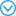The typical layout of power systems is experiencing significant change, due to the high penetration of renewable energy sources (RESs). The ongoing evaluation of power systems is expecting more detailed and accurate mathematical modeling approaches for RESs which are dominated by power electronics. Although modeling techniques based on state–space averaging (SSA) have traditionally been used to mathematically represent the dynamics of power systems, the performance of such a model-based system degrades under high switching frequency. The multi-frequency averaging (MFA)-based higher-index dynamic phasor modeling tool is proposed in this paper, which is entirely new and can provide better estimations of dynamics. Dynamic stability analysis is presented in this paper for the MFA-based higher-index dynamical model of single-stage single-phase (SSSP) grid-connected photovoltaic (PV) systems under different switching frequencies.

[J]. 太阳能学报, 2013, 34(11): 1860-1867.

MA Yahui, LI Xinran, LI Xiaoju, et al.

Research on the dynamic model of grid connected pv generation system

[J]. Acta Energiae Solaris Sinica, 2013, 34(11): 1860-1867.

[J]. 电力系统自动化, 2011, 35(2): 73-78.

ZHANG Jian, SUN Yuanzhang.

Impact of three-phase single-stage photovoltaic system on distribution network load modeling

[J]. Automation of Electric Power Systems, 2011, 35(2): 73-78.

[J]. 电力系统自动化, 2016, 40(8): 43-50.

LI Peiqiang, ZENG Xiaojun, HUANG Jiyuan, et al.

Equivalent modeling of grid-connected photovoltaic power generation systems for comprehensive load

[J]. Automation of Electric Power Systems, 2016, 40(8): 43-50.

[J]. 电网技术, 2020, 44(6): 2143-2150.

QU Xing, LI Xinran, SHENG Yifa, et al.

Research on equivalent modeling of PV generation system for generalized load

[J]. Power System Technology, 2020, 44(6): 2143-2150.

[J]. 中国电机工程学报, 2011, 31(34): 1-9.

WANG Haohuai, TANG Yong, HOU Junxian, et al.

Composition modeling and equivalence of an integrated power generation system of wind, photovoltaic and energy storage unit

[J]. Proceedings of the CSEE, 2011, 31(34): 1-9.

[J]. 中国电机工程学报, 2011, 31(10): 12-18.

LI Naiyong, LIANG Jun, ZHAO Yishu.

Research on dynamic modeling and stability of grid-connected photovoltaic power station

[J]. Proceedings of the CSEE, 2011, 31(10): 12-18.

[J]. 计算机仿真, 2015, 32(10): 140-143.

WANG Ruimiao, OUYANG Jinxin, GAO Jin, et al.

Analysis of short-circuit current characteristics of photovoltaic generation under three-phase short circuit

[J]. Computer Simulation, 2015, 32(10): 140-143.

[J]. 现代电力, 2013, 30(2): 57-63.

PEI Qinglei, WANG Liguo, LIU Lili, et al.

Analysis on dynamic characteristics of grid-connected PV inverter under power grid fault

[J]. Modern Electric Power, 2013, 30(2): 57-63.

[J]. 电力系统保护与控制, 2015, 43(7): 70-75.

XI Wei, ZHANG Zhe, CHEN Wei, et al.

Research on fault characteristic of the PV inverter

[J]. Power System Protection and Control, 2015, 43(7): 70-75.

[J]. 电力系统保护与控制, 2015, 43(1): 1-8.

YAN Kai, ZHANG Baohui, QU Jiping, et al.

Photovoltaic power system transient modeling and equivalents

[J]. Power System Protection and Control, 2015, 43(1): 1-8.

[J]. 办公自动化, 2020, 25(13): 29-31.

HUANG Pei.

The system parameter identification based on least squares

[J]. Office Informatization, 2020, 25(13): 29-31.

[J]. 西安交通大学学报, 2020, 54(4): 10-18.

WANG Zhiyu, WANG Bin, WANG Chaohui.

A parameter identification method for an equivalent circuit model of supercapacitor using nonlinear least squares

[J]. Journal of Xi'an Jiaotong University, 2020, 54(4): 10-18.

[J]. 电力系统及其自动化学报, 2013, 25(5): 116-120.

FU Bingbin, JIA Chunrong, YANG Changhai, et al.

Parameter identification of distributed photovoltaic power generation system

[J]. Proceedings of the CSU-EPSA, 2013, 25(5): 116-120.

/

 〈〉